# SSC EXAMS | Quantitative Aptitude Practice Questions (Day-24)

Dear Aspirants, Here we have given the Important SSC Exam 2019 Practice Test Papers. Candidates those who are preparing for SSC 2019 can practice these questions to get more confidence to Crack SSC 2019 Examination.

[WpProQuiz 5073]

1) Allowing 20%, and 15%, 10% and 30% successive discounts, the selling price of on article becomes 17136. Then the marked price will be.

a) 42,000

b) 32,000

c) 36,000

d) 40,000

2) Water is flowing at the rate of 10 km/h through a pipe of diameter 28 cm into a rectangular tank which is 100 m long, 88 m wide. The time taken in hours, for the rise in the level of water in the tank to be 14 cm  is—

a) 3hr

b) 2hr

c) 5hr

d) 1.75 hr.

3) The ratio of milk and water in mixtures of five containers are 1: 2, 2: 5, 3: 5, 2: 3 & 4: 7 respectively, in which container is the quantity of water, relative to milk maximum?

a) 2nd

b) 3rd

c) 4th

d) 5th

4) A man can row 8 km/h in still water. If the speed of the current is 4 km/hr. it takes 2 hours more in upstream than in the downstream for the same distance. The distance is:

a) 10 km

b) 12 km

c) 8 km

d) 2 km

5)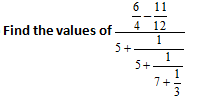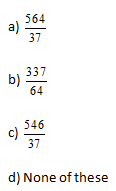6)  If x > 0, the minimum value of

a) 51

b) 50

c) 49

d) None of these

7) Two lamp posts are ‘x’ meters apart and the height of one is triple that of the other. If from middle point of the line joining their feet, on observer finds the angular elevations of their taps to be complementary, then the height (in meters of the shorter lamp post) is–

a) √3/x

b) x√3/6

c) 6x/√3

d) x/3√6

8) Find the equation of the straight line which makes an angle of 75o with the positive direction of x – axis and which meets on intercepts of length 6 on the negative direction of y – axis.

a) (√2 + 3)x – 6

b) (√2 – 3)x – 6

c) (√2 – 3)x + 6

d) (√2 ± 3)x ± 6

9) Roy bought a total of 12 fruits (Grapes, Pine and Apple) from the super market. He found that he required 2 (%) less to extract the same quantity of Juice as extracted from Grapes. If Roy has used the same number of grapes to make the blend, then which of the following correctly represents the percentage of grapes juice in the blend?

a) 40%

b) 35.355

c) 45.45%

d) 50%

10) Find the remainder of expression which is divided by 7?

N = 11×12 × 13 × 14 × 15 × 16 × 17 × 18 × 19 × 20 × 21 × 22.

a) 6

b) 7

c) 1

d) 0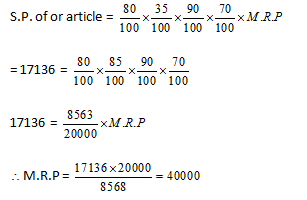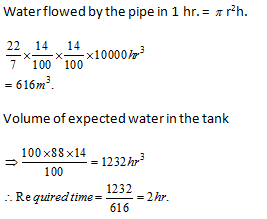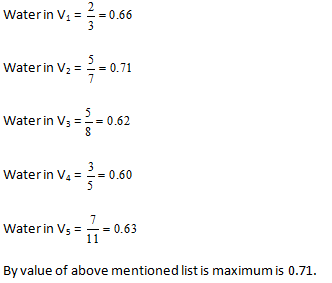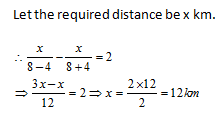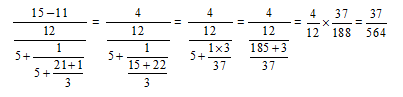Here the minimum value of x is 1.

28 + 2 – 2 + 111/24 – 2 + 2 – 9 = (256 + 101) / (16 – 9) = 357/7 = 51

From the given figure, we see that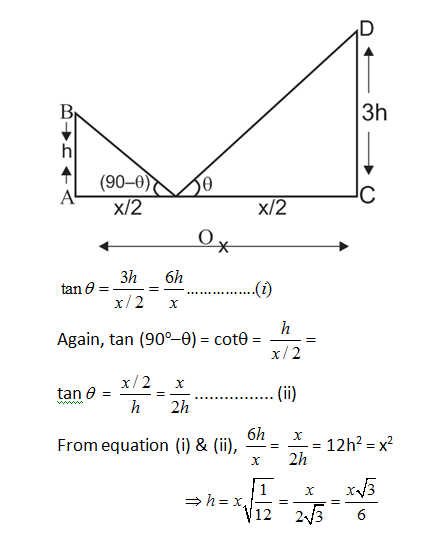∵ m = slope of the line = tan 75o = tan (45o + 30o)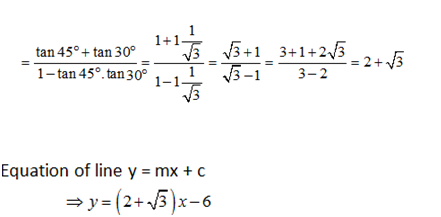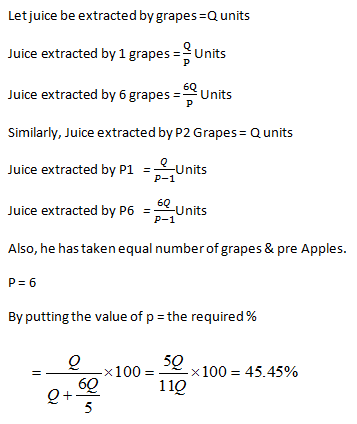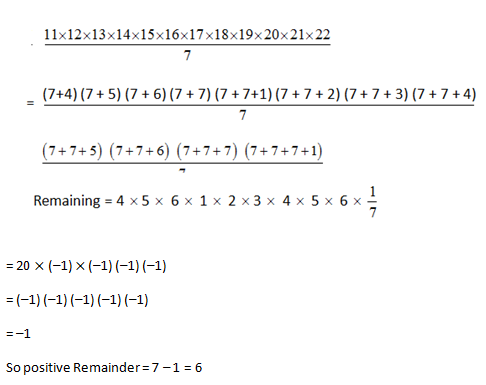### *******************

#### SSC CGL EXAMS 2019 |Quantitative Aptitude Practice Questions (Day-21)